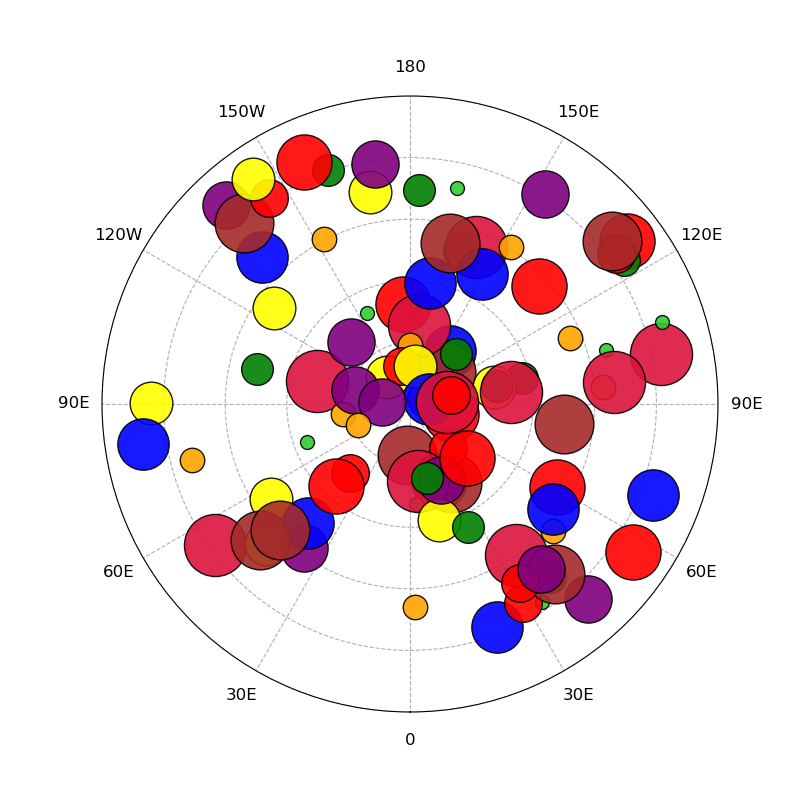# NCL_scatter_6.py#

This script illustrates the following concepts:
• Drawing a scatter plot with markers of different colors and sizes

• Drawing outlined and filled markers on a polar map plot

• Generating dummy data using “random”

• Changing the marker colors on a polar map plot

• Changing the marker sizes on a polar map plot

• Turning off y-axis labels

See following URLs to see the reproduced NCL plot & script:

Import packages:

```import numpy as np
import matplotlib.pyplot as plt
```

Create dummy data:

```numpoints = 100
lon = np.random.uniform(0, 360, numpoints)
lat = np.random.uniform(5, 90, numpoints)
```
```# Generate a figure
fig = plt.figure(figsize=(8, 8))

# Create axis with a polar projection
ax.set_ylim([0, 90])

# Change orientation of projection
ax.set_theta_zero_location("S")

# Create array of marker colors
colors = ("limegreen", "orange", "green", "red", "yellow", "purple", "blue",
"red", "brown", "crimson", "skyblue")

# Create array of marker sizes
bins = np.linspace(100, 2000, 10)

# Plot all points
# longitude points must be transformed from degrees to radians
# to be plotted on polar projection
for x in range(numpoints):
ax.scatter((np.pi / 180.0) * lon[x],
lat[x],
color=colors[x % 10],
s=bins[x % 10],
edgecolors='black',
linewidths=1,
alpha=0.9,
zorder=2)

# set the labels and locations of the angular gridlines
linelabels = ('0', '30E', '60E', '90E', '120E', '150E', '180', '150W', '120W',
'90E', '60E', '30E')
lines, labels = plt.thetagrids(range(0, 360, 30), linelabels, fontsize=12)

# Create distance between the x tick labels and the axis

# Set y-axis gridlines and turn off y-axis labels
ax.set_yticks([0, 20, 40, 60, 80, 100])
ax.set_yticklabels([])

# Make gridlines dashed
ax.grid(linestyle='--')

plt.show()
```Total running time of the script: (0 minutes 0.536 seconds)

Gallery generated by Sphinx-Gallery